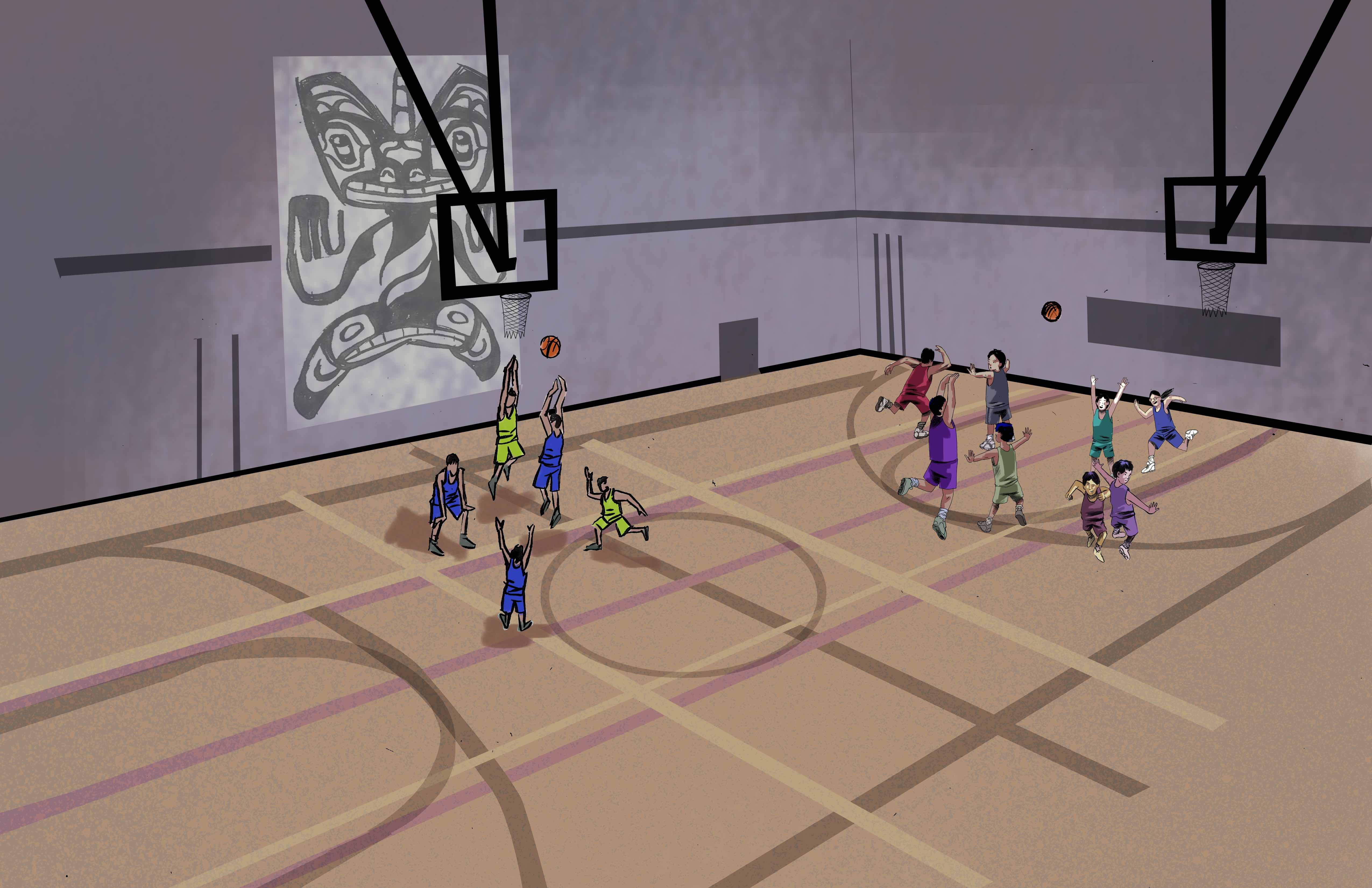## Section3.1Small Number and the Basketball Tournament - Classroom Guide

View the full story: Small Number and the Basketball Tournament

### Subsection3.1.1Mathematics

1. counting
2. patterns
3. mathematical games
4. mathematics and sports
5. mathematical thinking
6. combinatorics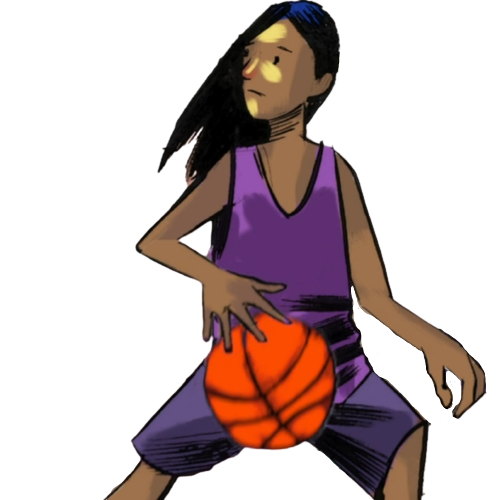### Subsection3.1.2Mathematical Vocabulary

• small, number, young, a lot, circle, big, older, Perfect Number, twice a week, after, first, half an hour, sometimes, algebra, mathematical games, Set, finding patterns, faster than anyone else, four basketball hoops, just a fraction of the court, the shortest boy in his group, very fast, Big Circle, the biggest boy on the team, a big half-court basketball tournament, each team, six players, only three players, on the court at one time, only five boys, the sixth player, tall, fast, join, finishing her math homework, tightly, very puzzled, the following day, beating a bit faster, in one voice, we can have twenty different teams, calculate things, all the time, in that case, ten different teams, runs away

### Subsection3.1.3Cultural Components

1. Indigenous:
3. The art presented in the film at 2:35 is inspired by the weavings of Skwetsimeltxw (Willard ‘Buddy’ Joseph) and Chepximiya Siyam (Janice George) displayed at Simon Fraser University’s Saywell Hall. Learn more about Salish Weavers Janice George and Buddy Joseph.
2. General:
1. What is your favourite team sport? Can you think of five mathematical questions related to your favourite sport? For example, “How many players are on each team?”, “What are the dimensions of the court or field?” or “How is the score calculated?”
2. Have you ever played the mathematical card game, Set? If yes, describe the game to your friends. If not, why don’t you try to learn it?
3. Check this out: Think about the number $6\text{.}$ We can divide the number $6$ by $1\text{,}$ $2\text{,}$ $3\text{,}$ and $6\text{.}$ Now observe that $1+2+3 = 6\text{.}$ This also works with the number $28\text{:}$ $1+2+4+7+14 = 28\text{.}$ The numbers $6$ and $28$ are called “perfect numbers”. Learn more about Perfect Numbers.
4. You can draw many circles on the surface of a ball. Some of those circles are bigger than others. The largest circles on the sphere are called “great circles” or “big circles”. Learn more about Great Circles.
5. Have you ever heard about the area of mathematics called “combinatorics”? Learn more about combinatorics from Kids Encyclopedia Facts and from Wikipedia.

### Subsection3.1.4Mathematical Observations (Video)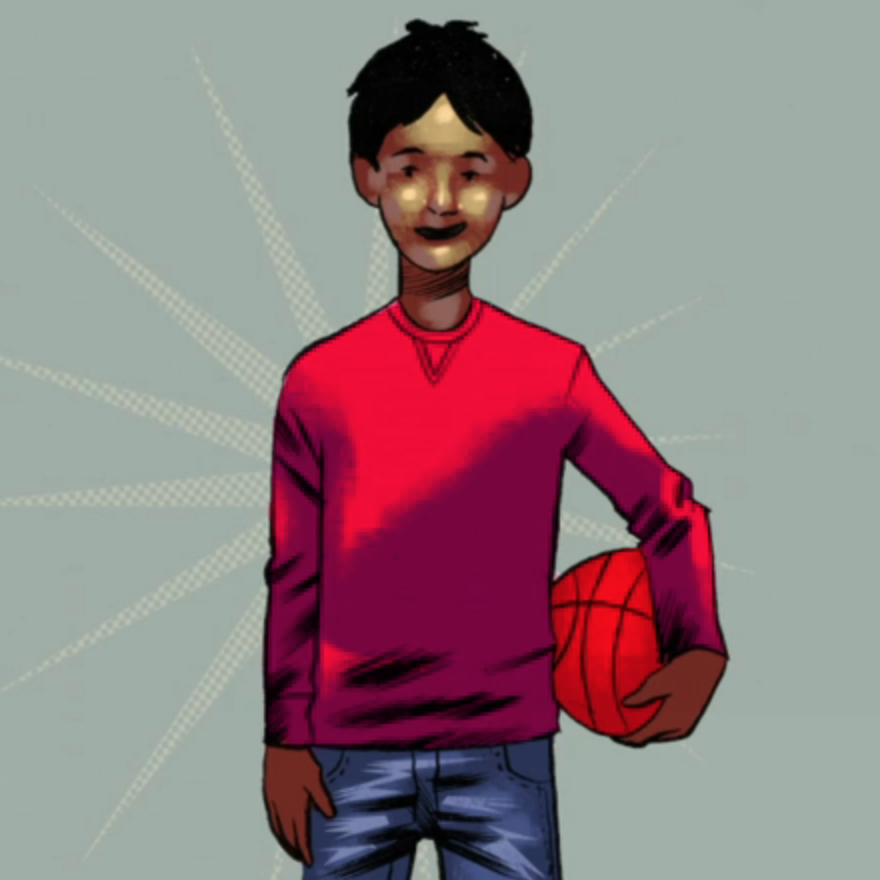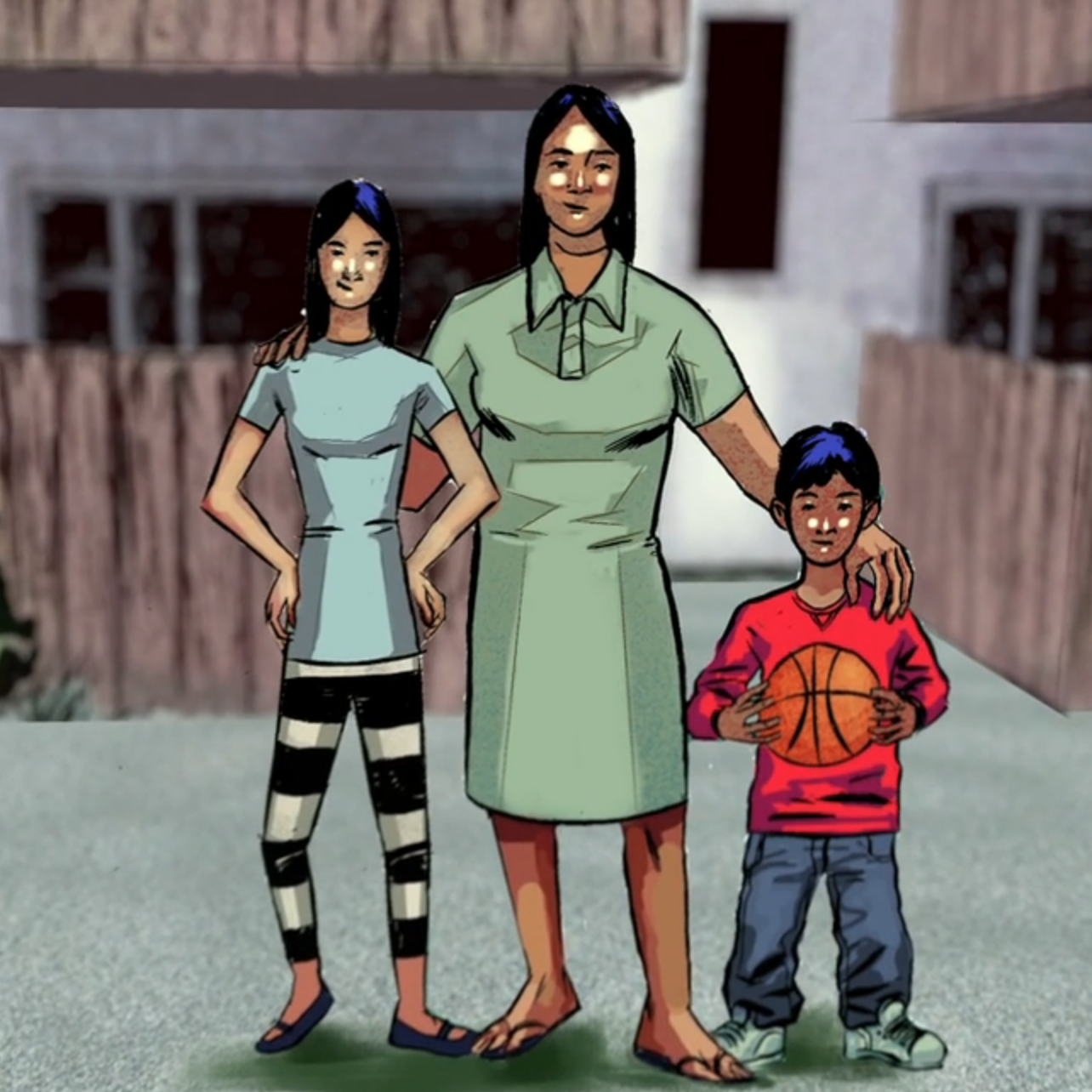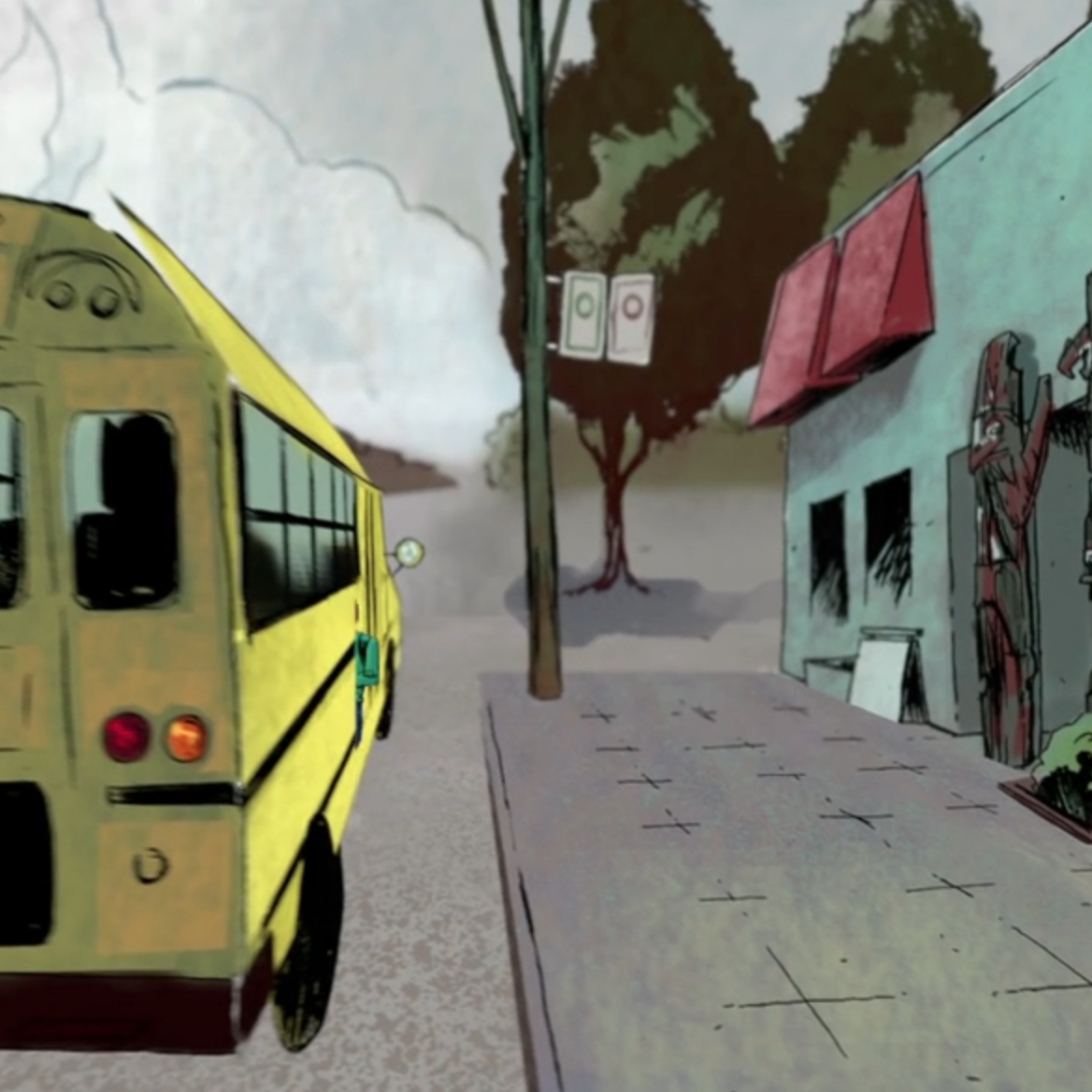Opening scene: Notice the shape of ball; the pattern on the ball; the pattern behind Small Number

0:06 - Notice the shape of the fence

0:15 - Notice the various shapes; straight lines; parallel lines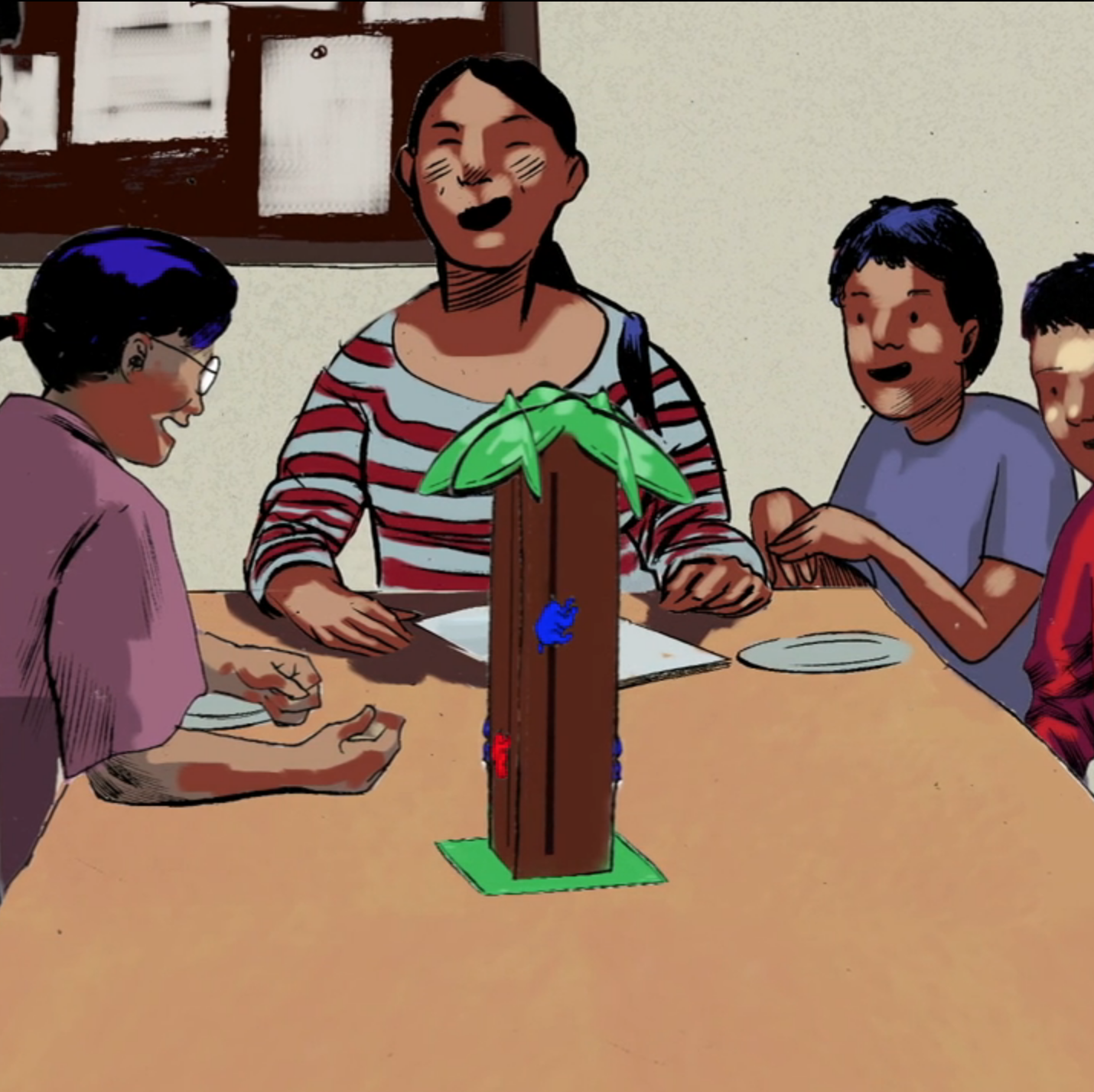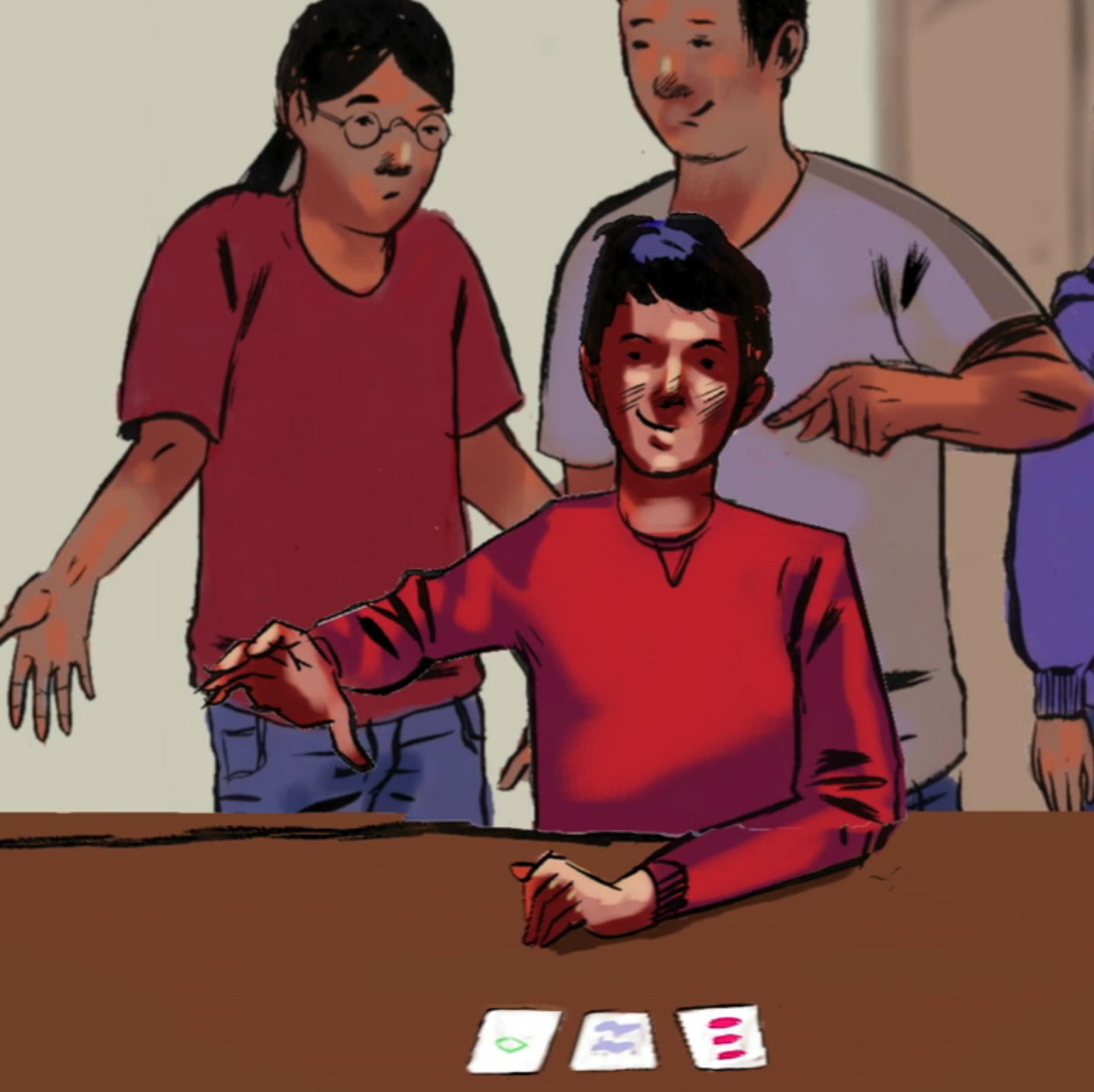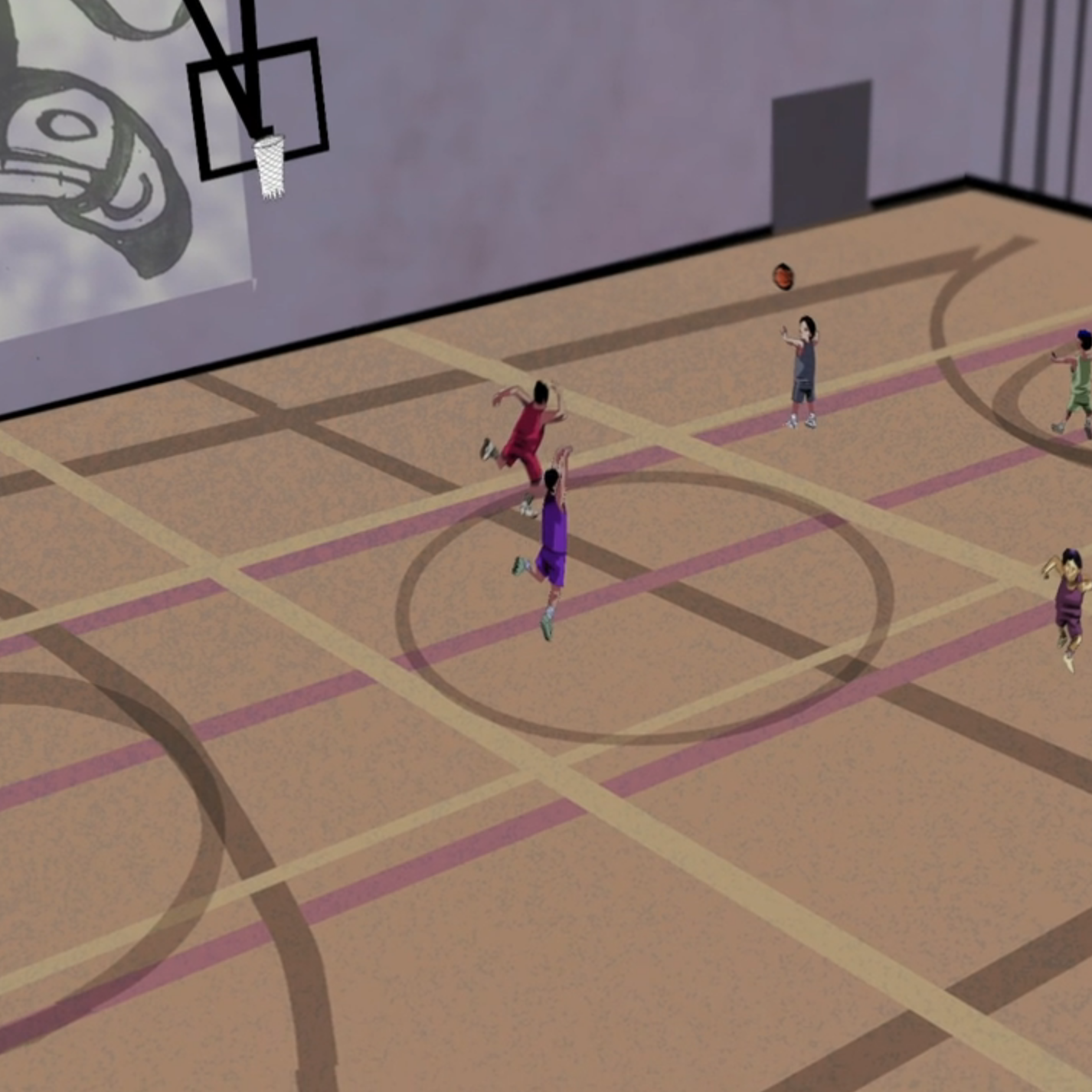0:29 - A version of the “Monkey Climbing Tree” game is on the table

0:33 - “Small Number’s favourite game is Set. He enjoys finding patterns faster than anyone else!”

0:39-0:50 - Consider the mathematics of a gym: patterns, shapes, and dimensions. “There are four basketball hoops in the gym, which allows each group of players to use just a fraction of the court.”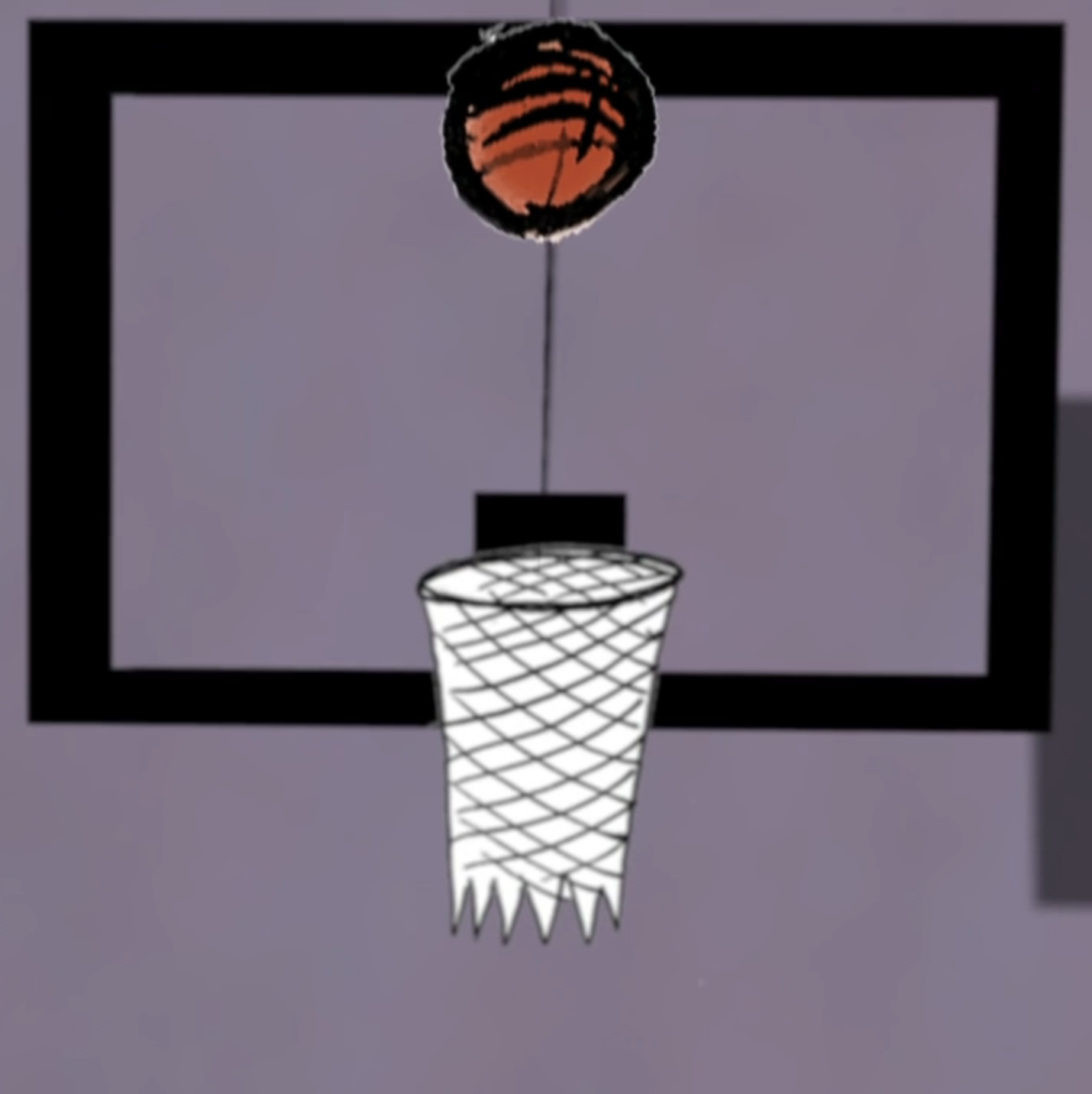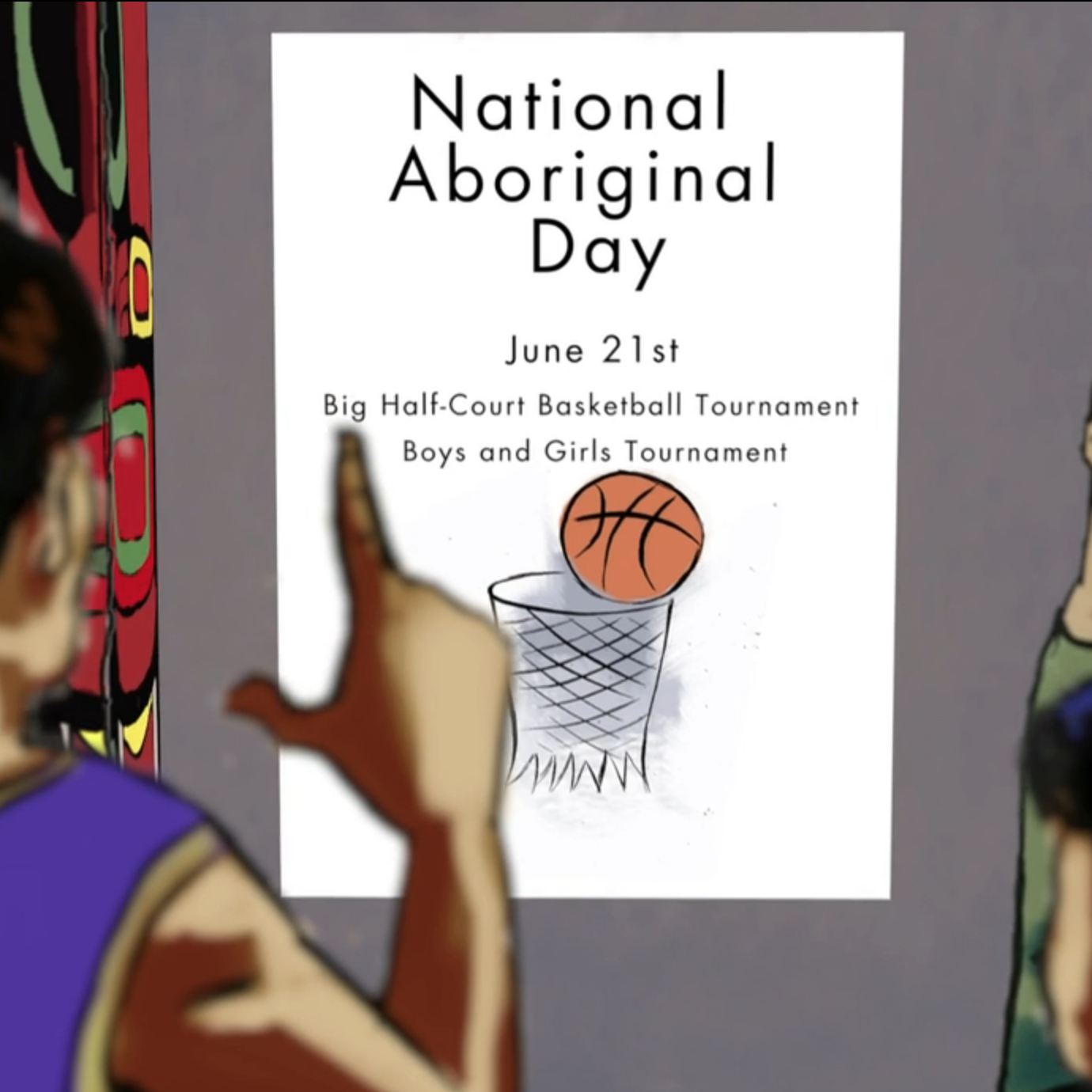0:50 - 1:00 - What is the shape of the curve that a thrown basketball follows?

1:08-1:14 - “Each team must have six players, but only three players are allowed on the court at one time.”

1:33 - Notice the geometry of the room: shapes, patterns, and reflections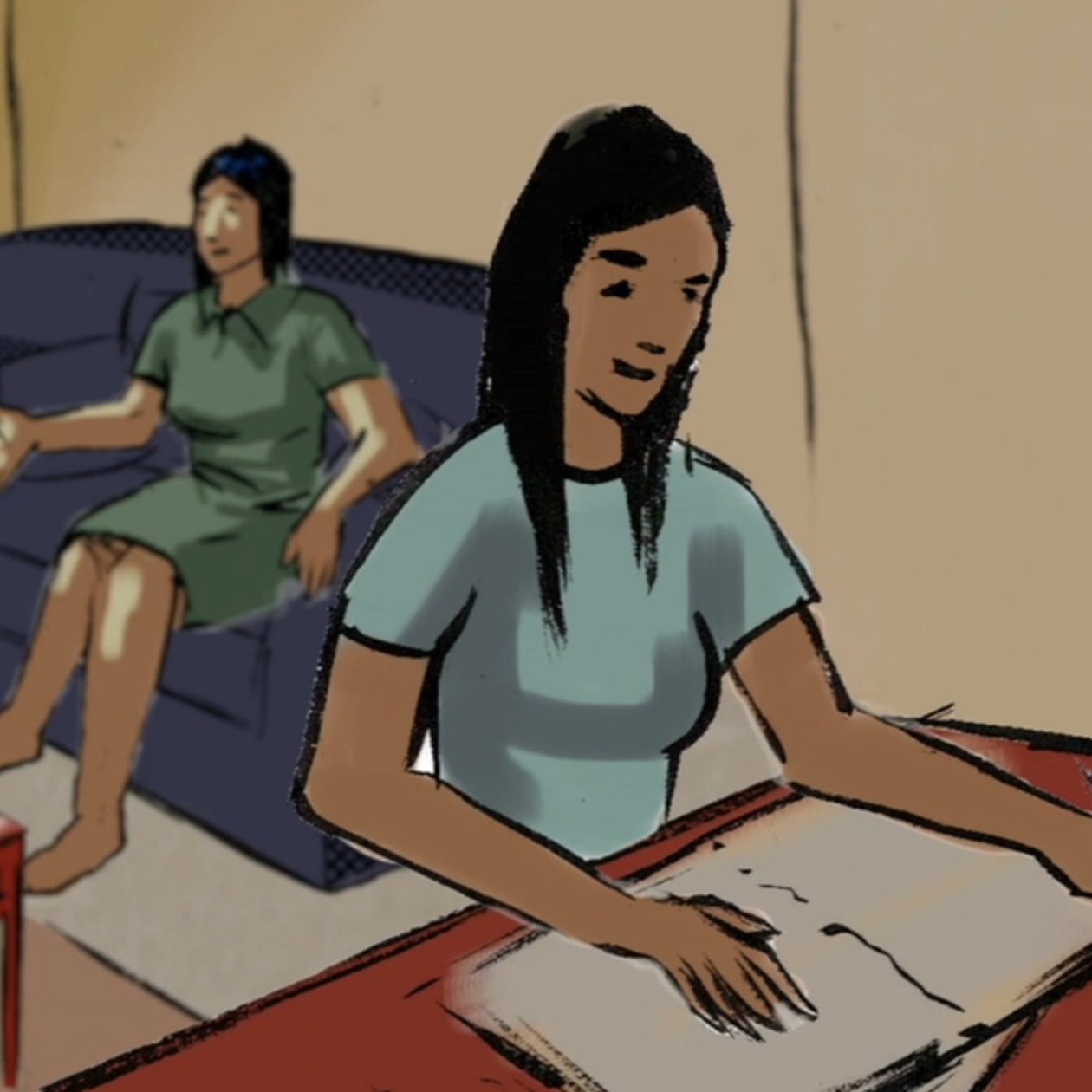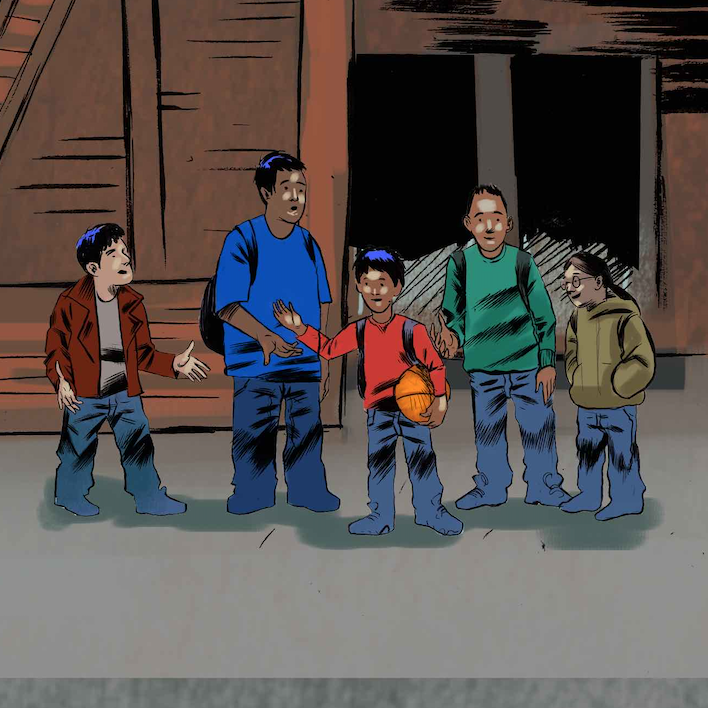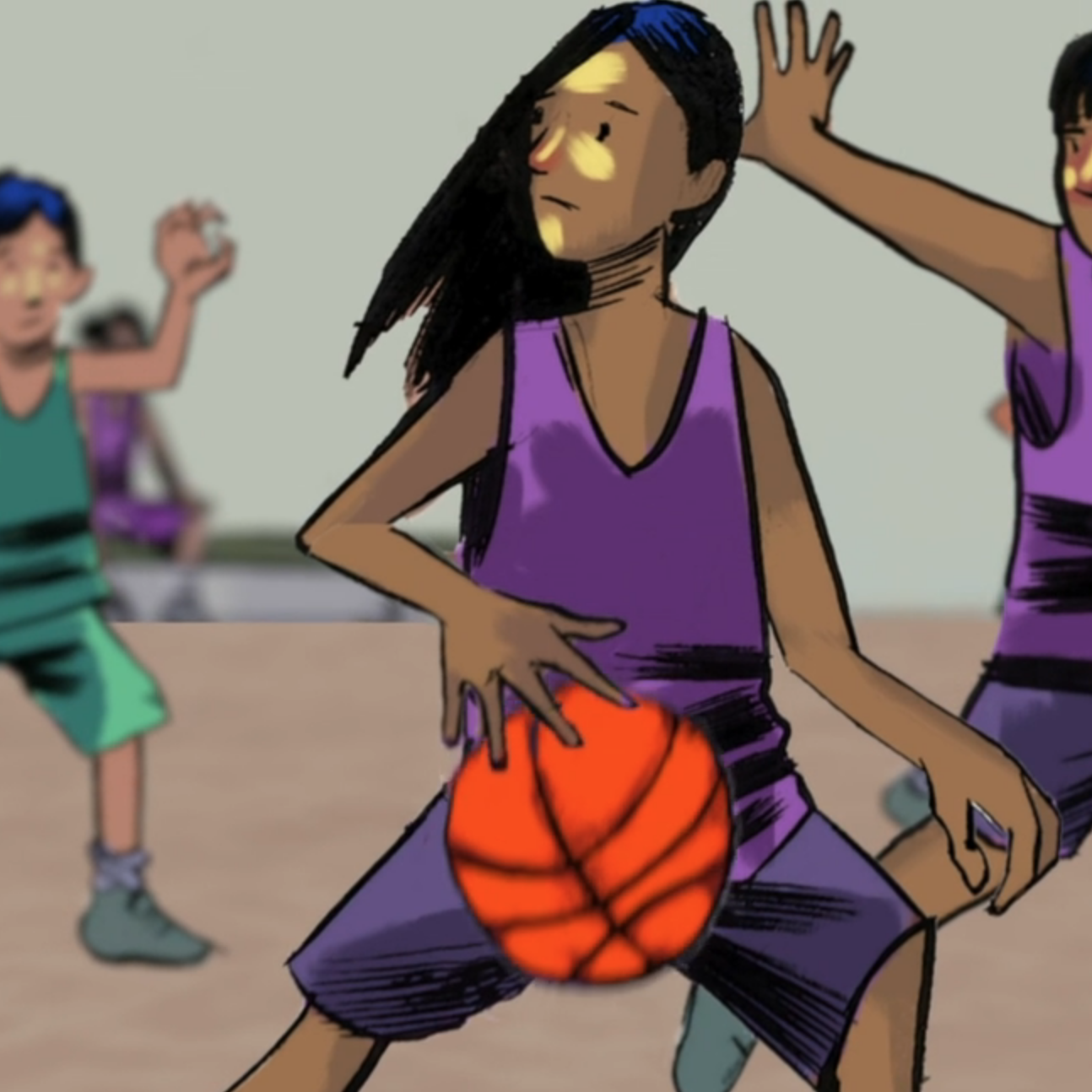1:56 - “She looks at Small Number’s sister Perfect Number, who is just finishing her math homework.”

2:06 - 2:18 - How many boys are there? Can you estimate each boys height? Notice the patterns in the background and the perspective.

2:23-2:32 - “With Perfect Number playing with us”, continues Small Number, “we can have twenty different teams on the court and nobody is ever going to get tired!”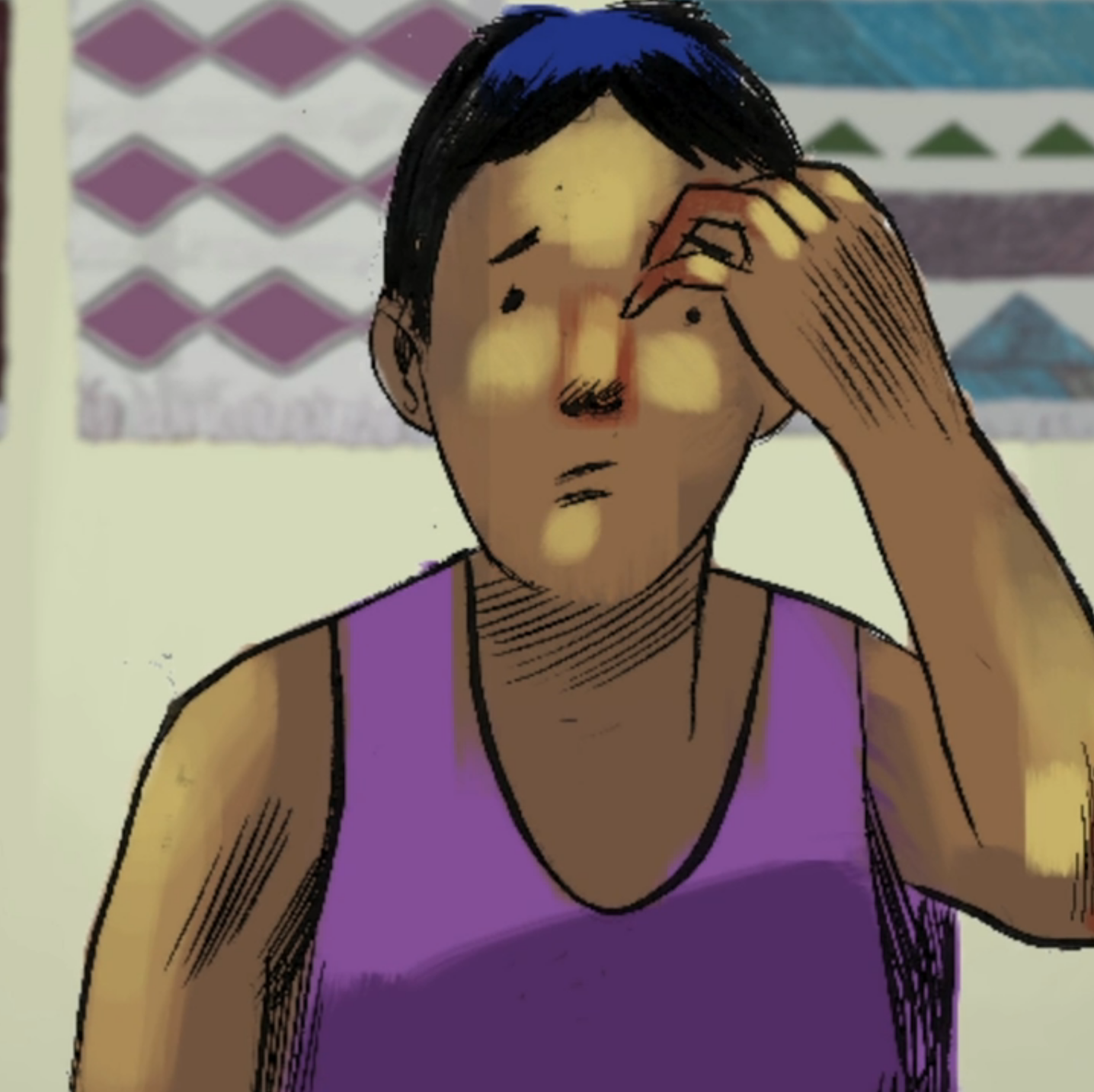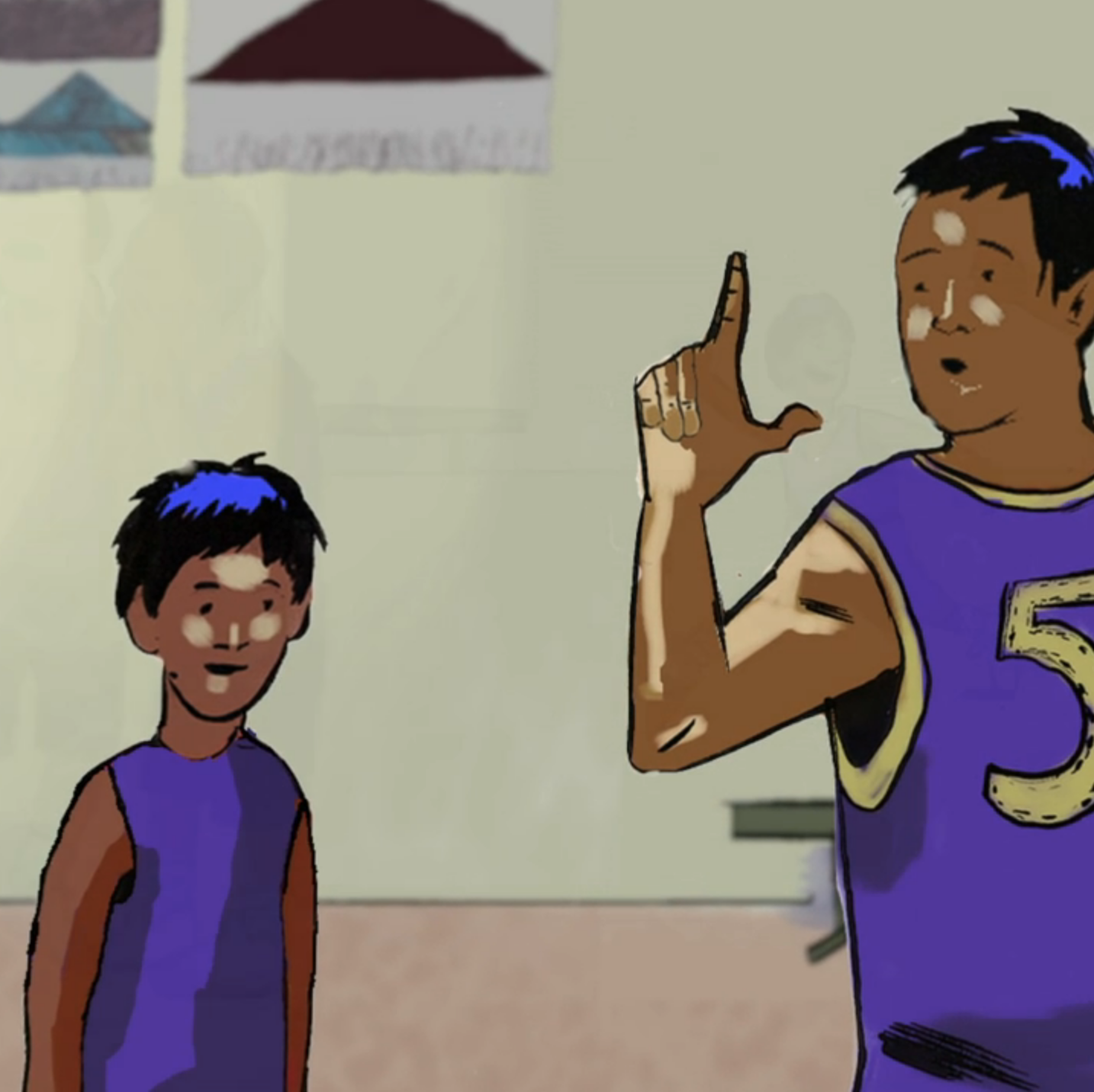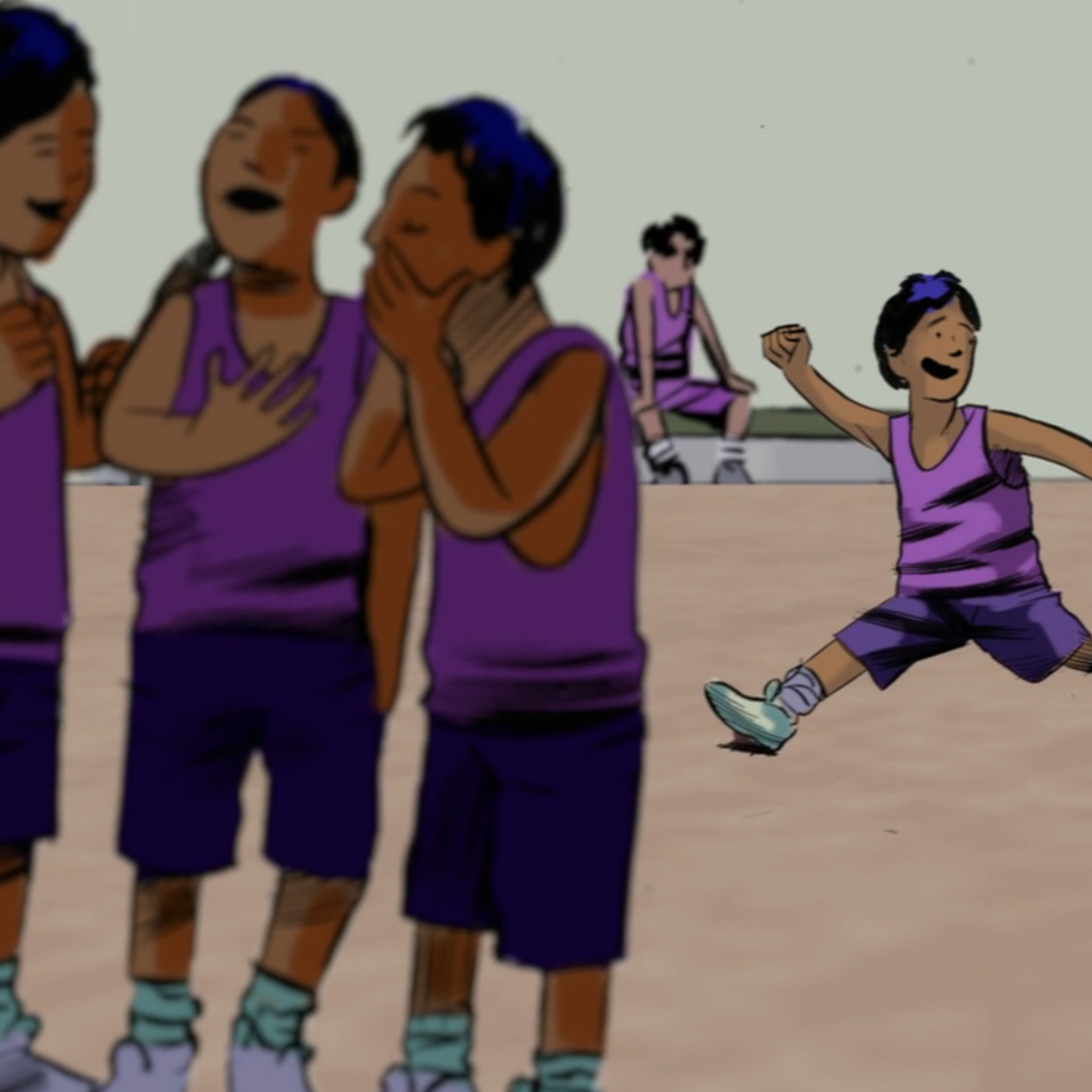2:34 - 2:38 Notice the patterns and the shapes on the art in the background

2:45 - “In that case, we can have ten different teams on the court.”

2:46 - 2:48 - How many people are there? How many groups of people? Notice the perspective.

### Subsection3.1.5Answer: How does Small Number know that if Perfect Number were to play with him and his friends, they would be able to have twenty different teams on the court during the tournament?

Big Circle: “How can you calculate things like that?”

Small Number thinks for a bit, and then starts explaining:

“There are six players on our team: Big Circle, Small Number, Perfect Number, Mos 1 , Nyânan 2 , and Naa 3 .”

“mos” is the Sliammon word for the number four. See Sliammon - First Voices. Here is the “Small Number and the Basketball Tournament” story in the Sliammon language.
“nyânan” is the Cree word for the number five. See How to speak Cree. Here is the “Small Number and the Basketball Tournament” story in the Cree language.
“naa” is the Blackfoot word for the number six. See Blackfoot Revitalization Project. Here is the “Small Number and the Basketball Tournament” story in the Blackfoot language.

“There can be only three people on the court at the same time. So what if we write down all of the possible teams and then count them?”

Big Circle: “This is difficult. I know that when I do things like this, I always forget something and I don’t get the right answer.”

Small Number smiles: “Yes, in mathematics it is always so easy to make a mistake, so we should be smart! Why don’t we list all of the teams in which two of us would play together?”

“OK” says Big Circle, while scratching his head.

Small Number takes a piece of paper, draws two vertical lines and a bunch of horizontal lines: “Let’s begin. Give me the team that we should start with.”

“Big Circle, Small Number, and… Perfect Number,” says Big Circle.

Small Number nods: “Great! Let me write that down.”

\begin{equation*} \begin{array}{c|c|c} \text{Big Circle} \ \ & \ \ \text{Small Number} \ \ & \ \ \text{Perfect Number}\\ \hline &&\\ \hline &&\\ \hline &&\\ \hline &&\\ \hline &&\\ \end{array} \end{equation*}

“OK, give me another team.”

“Big Circle, Perfect Number, and Small Number. But this time, Perfect Number will be a point guard and you will be a shooting guard” said Big Circle, hoping that Small Number didn’t notice that his voice trembled a bit each time he said Perfect Number’s name.

Small Number replies: “But those are still the same three people on the court. For our count of different teams, the role of each player is not important. For example, Big Circle, Small Number, and Mos will be another team. Like this:”

\begin{equation*} \begin{array}{c|c|c} \text{Big Circle} \ \ & \ \ \text{Small Number} \ \ & \ \ \text{Perfect Number}\\ \hline \text{Big Circle} & \text{Small Number} & \text{Mos}\\ \hline &&\\ \hline &&\\ \hline &&\\ \hline &&\\ \end{array} \end{equation*}

Big Circle thinks for a moment and then says: “Oh, I see. Then you replace Mos by Nyânan and Naa.”

“Yes, these are all of the possible teams with the two of us are on the court together,” agreed Small Number while completing the next two rows on his table:

\begin{equation*} \begin{array}{c|c|c} \text{Big Circle} \ \ & \ \ \text{Small Number} \ \ & \ \ \text{Perfect Number}\\ \hline \text{Big Circle} & \text{Small Number} & \text{Mos}\\ \hline \text{Big Circle} & \text{Small Number} & \text{Nyânan}\\ \hline \text{Big Circle} & \text{Small Number} & \text{Naa}\\ \hline &&\\ \hline &&\\ \end{array} \end{equation*}

“Now, let’s add all the teams when you and Perfect Number are on the court but I am not,” said Small Number:

\begin{equation*} \begin{array}{c|c|c} \text{Big Circle} \ \ & \ \ \text{Small Number} \ \ & \ \ \text{Perfect Number}\\ \hline \text{Big Circle} & \text{Small Number} & \text{Mos}\\ \hline \text{Big Circle} & \text{Small Number} & \text{Nyânan}\\ \hline \text{Big Circle} & \text{Small Number} & \text{Naa}\\ \hline \text{Big Circle} & \text{Perfect Number} & \text{Mos}\\ \hline \text{Big Circle} & \text{Perfect Number} & \text{Nyânan}\\ \hline \text{Big Circle} & \text{Perfect Number} & \text{Naa}\\ \hline &&\\ \hline &&\\ \end{array} \end{equation*}

Small Number looks up at his friend: “What should we do next?”

“Now we should add all of the teams when I am on the court but neither Perfect Number nor you are,” replies Big Circle.

“You got it! Here is the list of all the teams with you on the court,” Small number is talking and writing at the same time:

\begin{equation*} \begin{array}{c|c|c|c} 1 & \text{Big Circle} \ \ & \ \ \text{Small Number} \ \ & \ \ \text{Perfect Number}\\ \hline 2 & \text{Big Circle} & \text{Small Number} & \text{Mos}\\ \hline 3 & \text{Big Circle} & \text{Small Number} & \text{Nyânan}\\ \hline 4 & \text{Big Circle} & \text{Small Number} & \text{Naa}\\ \hline 5 & \text{Big Circle} & \text{Perfect Number} & \text{Mos}\\ \hline 6 & \text{Big Circle} & \text{Perfect Number} & \text{Nyânan}\\ \hline 7 & \text{Big Circle} & \text{Perfect Number} & \text{Naa}\\ \hline 8 & \text{Big Circle} & \text{Mos} & \text{Nyânan}\\ \hline 9 & \text{Big Circle} & \text{Mos} & \text{Naa}\\ \hline 10 & \text{Big Circle} & \text{Nyânan} & \text{Naa}\\ \end{array} \end{equation*}

“You said that there would be ten different teams if I am on the court. Let me count: one, two, three… ten! How did you know this before writing anything down, Small Number?”

“I’ll tell you later. Let me continue writing down all of the different teams. First I’ll add all of the teams when I am on the court but you are not.”

\begin{equation*} \begin{array}{c|c|c|c} 1 & \ \ \text{Big Circle} \ \ & \ \ \text{Small Number} \ \ & \ \ \text{Perfect Number}\\ \hline 2 & \text{Big Circle} & \text{Small Number} & \text{Mos}\\ \hline 3 & \text{Big Circle} & \text{Small Number} & \text{Nyânan}\\ \hline 4 & \text{Big Circle} & \text{Small Number} & \text{Naa}\\ \hline 5 & \text{Big Circle} & \text{Perfect Number} & \text{Mos}\\ \hline 6 & \text{Big Circle} & \text{Perfect Number} & \text{Nyânan}\\ \hline 7 & \text{Big Circle} & \text{Perfect Number} & \text{Naa}\\ \hline 8 & \text{Big Circle} & \text{Mos} & \text{Nyânan}\\ \hline 9 & \text{Big Circle} & \text{Mos} & \text{Naa}\\ \hline 10 & \text{Big Circle} & \text{Nyânan} & \text{Naa}\\ \hline 11 & \text{Small Number} & \text{Perfect Number} & \text{Mos}\\ \hline 12 & \text{Small Number} & \text{Perfect Number} & \text{Nyânan}\\ \hline 13 & \text{Small Number} & \text{Perfect Number} & \text{Naa}\\ \hline 14 & \text{Small Number} & \text{Mos} & \text{Nyânan}\\ \hline 15 & \text{Small Number} & \text{Mos} & \text{Naa}\\ \hline 16 & \text{Small Number} & \text{Nyânan} & \text{Naa}\\ \end{array} \end{equation*}

“Now write all of the teams when Perfect Number is on the court, but neither of us are.” Big Circle was becoming impatient.

“Yes, and then there will one more team without you, me, or my sister!”

\begin{equation*} \begin{array}{c|c|c|c} 1 & \ \ \text{Big Circle} \ \ & \ \ \text{Small Number} \ \ & \ \ \text{Perfect Number}\\ \hline 2 & \text{Big Circle} & \text{Small Number} & \text{Mos}\\ \hline 3 &\text{Big Circle} & \text{Small Number} & \text{Nyânan}\\ \hline 4 &\text{Big Circle} & \text{Small Number} & \text{Naa}\\ \hlineno too much 5 &\text{Big Circle} & \text{Perfect Number} & \text{Mos}\\ \hline 6 &\text{Big Circle} & \text{Perfect Number} & \text{Nyânan}\\ \hline 7 &\text{Big Circle} & \text{Perfect Number} & \text{Naa}\\ \hline 8 &\text{Big Circle} & \text{Mos} & \text{Nyânan}\\ \hline 9 &\text{Big Circle} & \text{Mos} & \text{Naa}\\ \hline 10 &\text{Big Circle} & \text{Nyânan} & \text{Naa}\\ \hline 11&\text{Small Number} & \text{Perfect Number} & \text{Mos}\\ \hline 12 &\text{Small Number} & \text{Perfect Number} & \text{Nyânan}\\ \hline 13 &\text{Small Number} & \text{Perfect Number} & \text{Naa}\\ \hline 14 &\text{Small Number} & \text{Mos} & \text{Nyânan}\\ \hline 15 &\text{Small Number} & \text{Mos} & \text{Naa}\\ \hline 16 &\text{Small Number} & \text{Nyânan} & \text{Naa}\\ \hline 17 &\text{Perfect Number} & \text{Mos} & \text{Nyânan}\\ \hline 18 &\text{Perfect Number} & \text{Mos} & \text{Naa}\\ \hline 19 &\text{Perfect Number} & \text{Nyânan} & \text{Naa}\\ \hline 20 &\text{Mos} & \text{Nyânan} & \text{Naa}\\ \end{array} \end{equation*}

“Twenty different teams altogether!”

### Subsection3.1.6Discussion/Activities

The purpose of this activity is to introduce very young learners to the notions of permutations and combinations, i.e. the distinction between “ordering things” and “not-ordering things”.

Recall that finding the number of permutations and the number of combinations is related to counting in how many times we can choose $r$ out of $n$ given objects:

Permutation Rule:

Order matters. For example, $(1, 2, 3)\text{,}$ $(2,1,3)\text{,}$ and $(2,4,5)$ are three different 3-permutations of the set $\{1, 2, 3, 4, 5\}\text{.}$

Combination Rule:

Order does not matter. For example, $\{1, 2, 3\}$ and $\{2, 4, 5\}$ are two different 3–combinations of the set $\{1, 2, 3, 4, 5\}\text{.}$ But, $\{1, 2, 3\}$ and $\{2, 1, 3\}$ represent the same 3-combinations of the set $\{1, 2, 3, 4, 5\}\text{.}$

Observe how round brackets $( · , · , · )$ are used to indicate that order matters and how curly brackets $\{ · , · , · \}$ are used to indicate that order does not matter.

• Part 1 - Permutations - Order Matters

Put three chairs beside each other. Clearly label them: 1, 2, and 3. You may wish to use chairs of different colours.

Prepare a chart like the one below (or draw it on the board) with 24 blank rows:

\begin{equation*} \begin{array}{c|c|c|cc} \# \ \ &\ \ \text{Chair 1} \ \ &\ \ \text{Chair 2} \ \ &\ \ \text{Chair 3} \ \ \\ \hline &&&\\ \hline &&&\\ \hline &&&\\ \end{array} \end{equation*}

• Four students will perform the activity. (Let’s call these students Alice, Bob, Carol, and Dave.)
• Two students will record the different stages of the activity into the chart. (Erin and Frank)
• Three students will act as witnesses. 4  (Grace, Heidi, and Ivan)

Explain the activity:

• We will count how many different ways Alice, Bob, Carol, and Dave can sit on the three chairs. Obviously, we have four people and three chairs, so one person will have to wait for their turn.
• Erin and Frank will fill out the chart so that we do not lose count.
• Grace, Heidi, and Ivan will be the witnesses of what is going on. They will observe everything carefully and later on they will tell us in their own words what happened.

“Do you have any questions? Can we begin?”

To Alice, Bob, Carol and Dave: “Alice, please sit on chair 1, Bob you sit on chair 2, and Carol you sit on chair 3. Dave, can you please stay standing.”

To Erin and Frank: “Erin, please write ‘Alice’ under ‘chair 1’, ‘Bob’ under ‘chair 2’ and ‘Carol’ under ‘chair 3’. Frank, please write the number ‘1’ under the number sign.”

\begin{equation*} \begin{array}{c|c|c|cc} \# \ \ &\ \ \text{Chair 1} \ \ &\ \ \text{Chair 2} \ \ &\ \ \text{Chair 3} \ \ \\ \hline 1&\text{Alice}&\text{Bob}&\text{Carol}\\ \hline &&&\\ \hline &&&\\ \end{array} \end{equation*}

To the witnesses: “Remember that we started with Alice sitting on chair 1, Bob sitting on chair 2, and Carol sitting on chair 3.”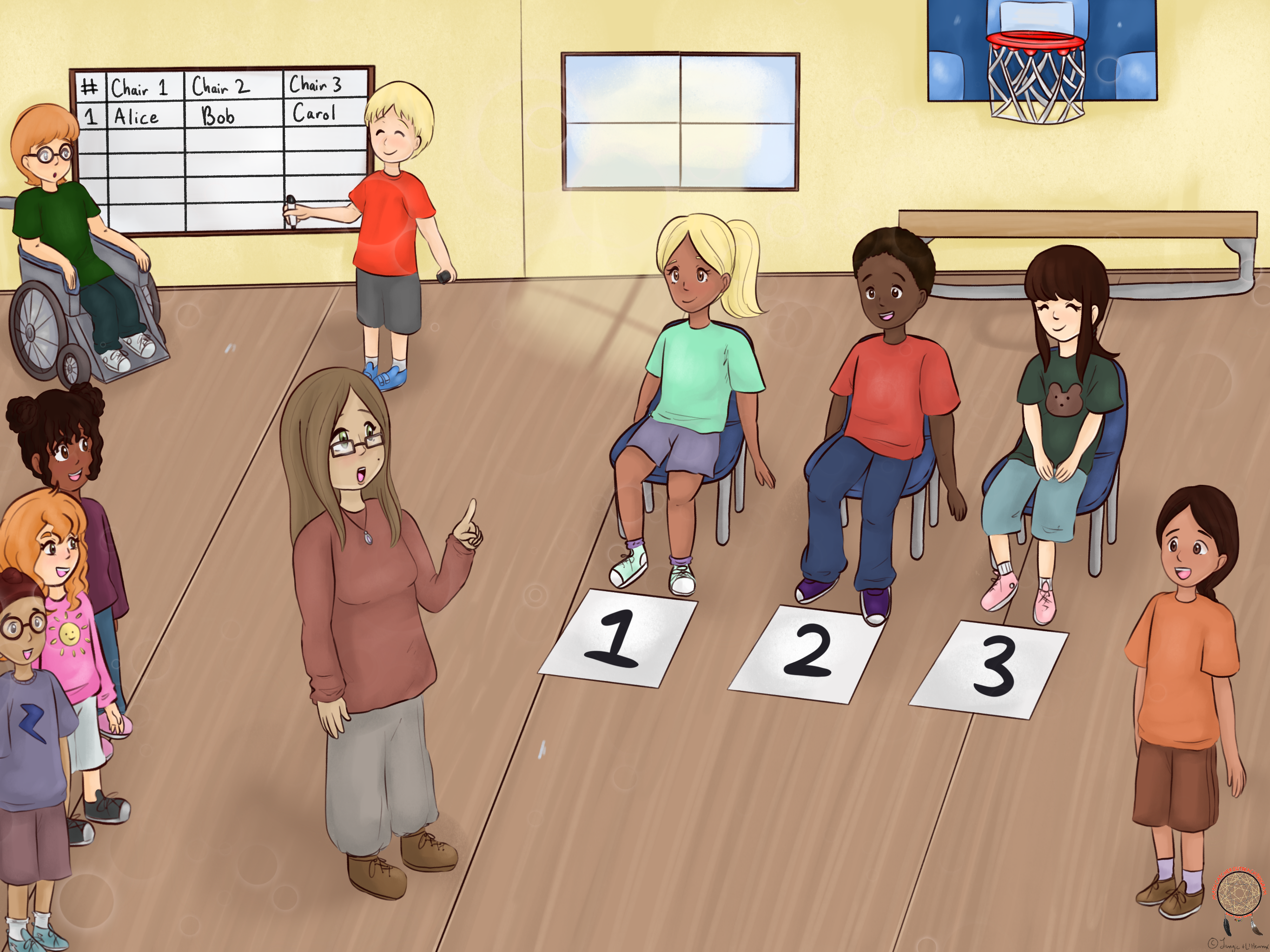To Alice Bob, and Carol: “Alice, please stay on chair 1. Bob and Carol, please exchange your chairs.” Bob and Carol switch their places.

To Erin and Frank: “Erin, please write ‘Alice’ under ‘chair 1’, ‘Carol’ under ‘chair 2’ and ‘Bob’ under ‘chair 3’. Frank, please write the number ‘2’ under the number sign.”

\begin{equation*} \begin{array}{c|c|c|cc} \# \ \ &\ \ \text{Chair 1} \ \ &\ \ \text{Chair 2} \ \ &\ \ \text{Chair 3} \ \ \\ \hline 1&\text{Alice}&\text{Bob}&\text{Carol}\\ \hline 2&\text{Alice}&\text{Carol}&\text{Bob}\\ \hline &&&\\ \end{array} \end{equation*}

To the witnesses: “Remember that I asked Alice to stay on chair 1, and that I asked Bob and Carol to switch their places.”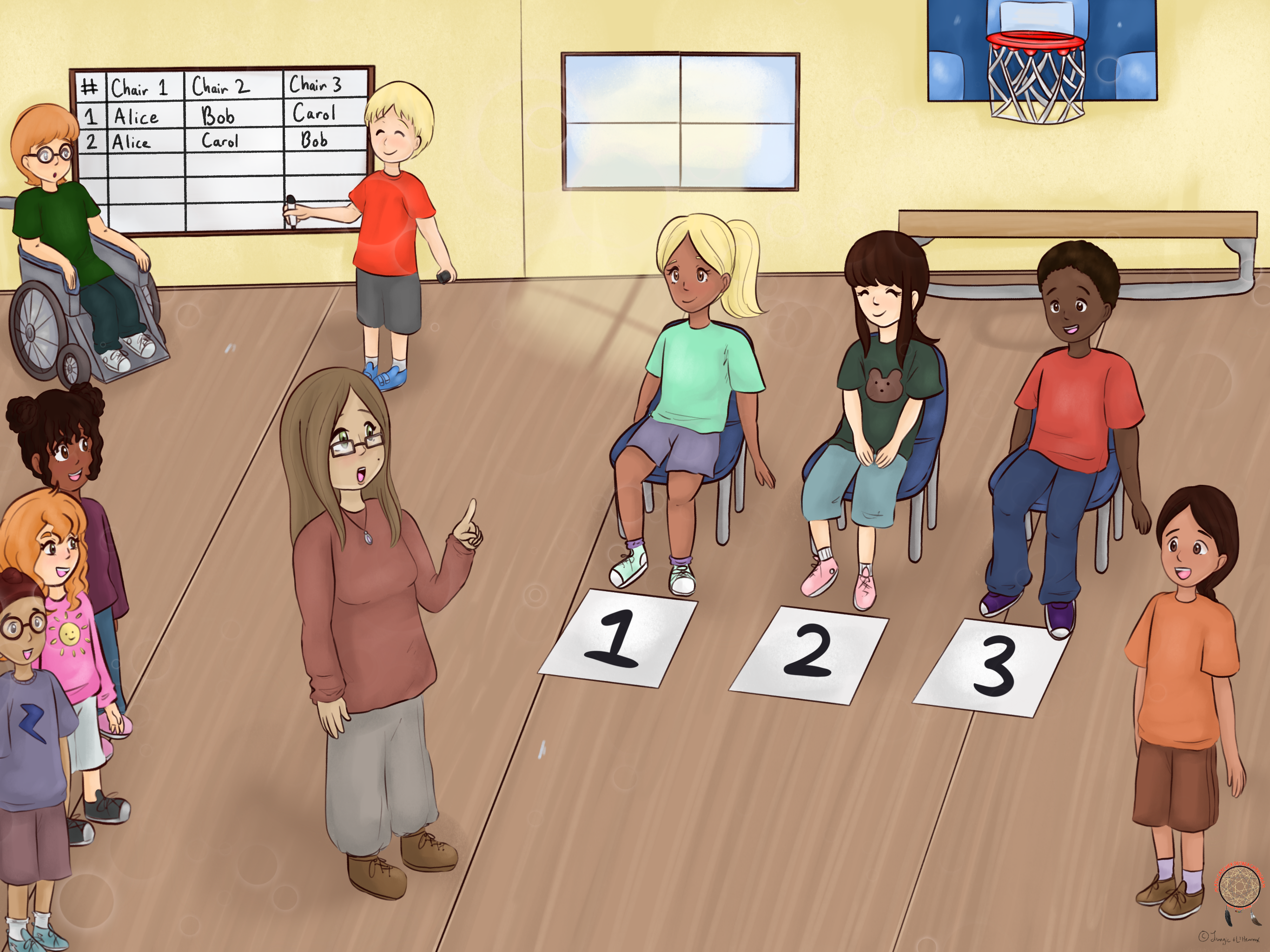To the class: “It is so easy to make a mistake when we count, so we always need to be very careful. What helps me to avoid mistakes is to count in some kind of order. I’d suggest that our strategy here be to first count all of the cases when Alice sits on chair 1. Do you agree?” [Class: “Yes, we agree!”]

To the class: “Thanks! OK, what should we do next?” [The idea is that Dave takes Carol’s seat. Maybe a student will suggests this, but maybe the teacher will need to help.]

To Dave and Carol: “Dave, please take Carol’s place. Carol, thank you, you will be standing for a little while.” [Recall that Alice is already sitting on chair 1 and that Bob is on chair 3.]

To Erin and Frank: “What should you write on the chart now?”

Frank: “I’ll write the number 3.”

Erin writes:

\begin{equation*} \begin{array}{c|c|c|cc} \# \ \ &\ \ \text{Chair 1} \ \ &\ \ \text{Chair 2} \ \ &\ \ \text{Chair 3} \ \ \\ \hline 1&\text{Alice}&\text{Bob}&\text{Carol}\\ \hline 2&\text{Alice}&\text{Carol}&\text{Bob}\\ \hline 3&\text{Alice}&\text{Dave}&\text{Bob}\\ \hline &&&\\ \end{array} \end{equation*}

To Erin and Frank: “Excellent job, Erin and Frank!”

To the class: “What should we do now? Don’t forget, our strategy is to keep Alice sitting on chair 1 for as long as possible.”

A student: “Now, Bob should stand up and Carol should take his seat.” This will lead to two more cases and Erin and Frank would produce the following chart:

\begin{equation*} \begin{array}{c|c|c|cc} \# \ \ &\ \ \text{Chair 1} \ \ &\ \ \text{Chair 2} \ \ &\ \ \text{Chair 3} \ \ \\ \hline 1&\text{Alice}&\text{Bob}&\text{Carol}\\ \hline 2&\text{Alice}&\text{Carol}&\text{Bob}\\ \hline 3&\text{Alice}&\text{Dave}&\text{Bob}\\ \hline 4&\text{Alice}&\text{Bob}&\text{Dave}\\ \hline 5&\text{Alice}&\text{Carol}&\text{Dave}\\ \hline 6&\text{Alice}&\text{Dave}&\text{Carol}\\ \hline &&&\\ \end{array} \end{equation*}

This is the key moment of this activity. Students need to realize the following:

1. The number of different cases with Alice sitting on chair 1 equals to six.
2. Another person, say Bob, should sit on chair 1.

Now, with Bob sitting on chair 1, ask the class: “What do you think, how many different cases will Erin and Frank complete with Bob sitting on chair 1?”

$\color{blue}{Script}$ $\color{blue}{1:}$ A student, say, Jane, answers, “Six!”

To Jane: “This is an excellent idea, Jane! But please explain to all of us your reasoning.”

Jane points to the chart: “If we switch Alice’s name and Bob’s name on the chart, we will have all cases with Bob sitting on chair 1. So six cases all together!”

OR

Jane points to chair 1: “It does not matter who is sitting on chair 1. It will always the same number of cases: six!”

OR

Jane points to chairs 2 and 3: “We still have three people for chairs 2 and 3. Their names do not matter. So the number of cases will be: six!”

$\color{blue}{Script}$ $\color{blue}{2:}$ No student comes up with the right answer. Encourage discussion. Lead students towards one of the “Jane’s answers” from Script 1.

$\color{blue}{Script}$ $\color{blue}{3:}$ Repeat the activity with Bob sitting on chair 1 and Alice, Carol, and Dave taking turns on chairs 2 and 3. Erin and Frank should record all of the cases. Ask the class to observe that the number of cases is six. Ask: “Are you surprised?”

Once when the class is convinced that, regardless who is sitting on chair 1, the number of cases is always six ask: “What do you think that the final answer should be?” If necessary, lead the class to conclusion that the answer is $4\times6 = 24\text{.}$

Ask for questions. Thank Alice, Bob, Carol, Dave, Erin and Frank. Ask the witnesses to describe what happened during the activity.

• Part 2 - Combinations - Order Does Not Matter

Mark a spot in the classroom.

Prepare a chart like the one below (or draw it on the board) with 4 blank rows:

\begin{equation*} \begin{array}{c|c} \text{Team #} \ \ \ &\ \ \hspace{1cm}\text{Players}\hspace{1cm} \ \ \\ \hline & \\ \hline & \\ \hline & \\ \hline & \\ \end{array} \end{equation*}

• Four students will perform the activity. (Let’s call these students Alice, Bob, Carol, Dave,)
• Two students will record the different stages of the activity in the chart. (Erin and Frank)
• Three students will act as witnesses. (Grace, Heidi, and Ivan)

Explain the activity:

• We will count how many different ways Alice, Bob, Carol, and Dave can form a team of three players. Obviously, we have four people, so each time one person will not be in the team.
• Erin and Frank will fill out this chart so that we do not lose count.
• Grace, Heidi, and Ivan will be the witnesses of what is going on. They will observe everything carefully and later they will tell us in their own words what happened.

“Do you have any questions? Can we begin?”

To Alice, Bob, Carol and Dave: “Alice, Bob, and Carol you will be Team 1. Please come forward and stand around the marked spot. Dave, please stay where you are”

To Erin and Frank: “Erin, please write ‘Alice, Bob, Carol’ under ‘Players’. Frank, please write the number ‘1’ under ‘Team’”

\begin{equation*} \begin{array}{c|c} \text{Team #} \ \ \ &\ \ \hspace{1cm}\text{Players}\hspace{1cm} \ \ \\ \hline 1&\text{Alice, Bob, Carol} \\ \hline & \\ \hline & \\ \hline & \\ \end{array} \end{equation*}

To the class: “Please listen carefully to this question: If Erin writes the names in a different order, would that be the same team or a different team?” [The class consensus should be: “Yes, that would be the same team!”]

To the witnesses: “Remember that we agreed that Alice, Bob, and Carol form the same team regardless of which order we write or say their names.”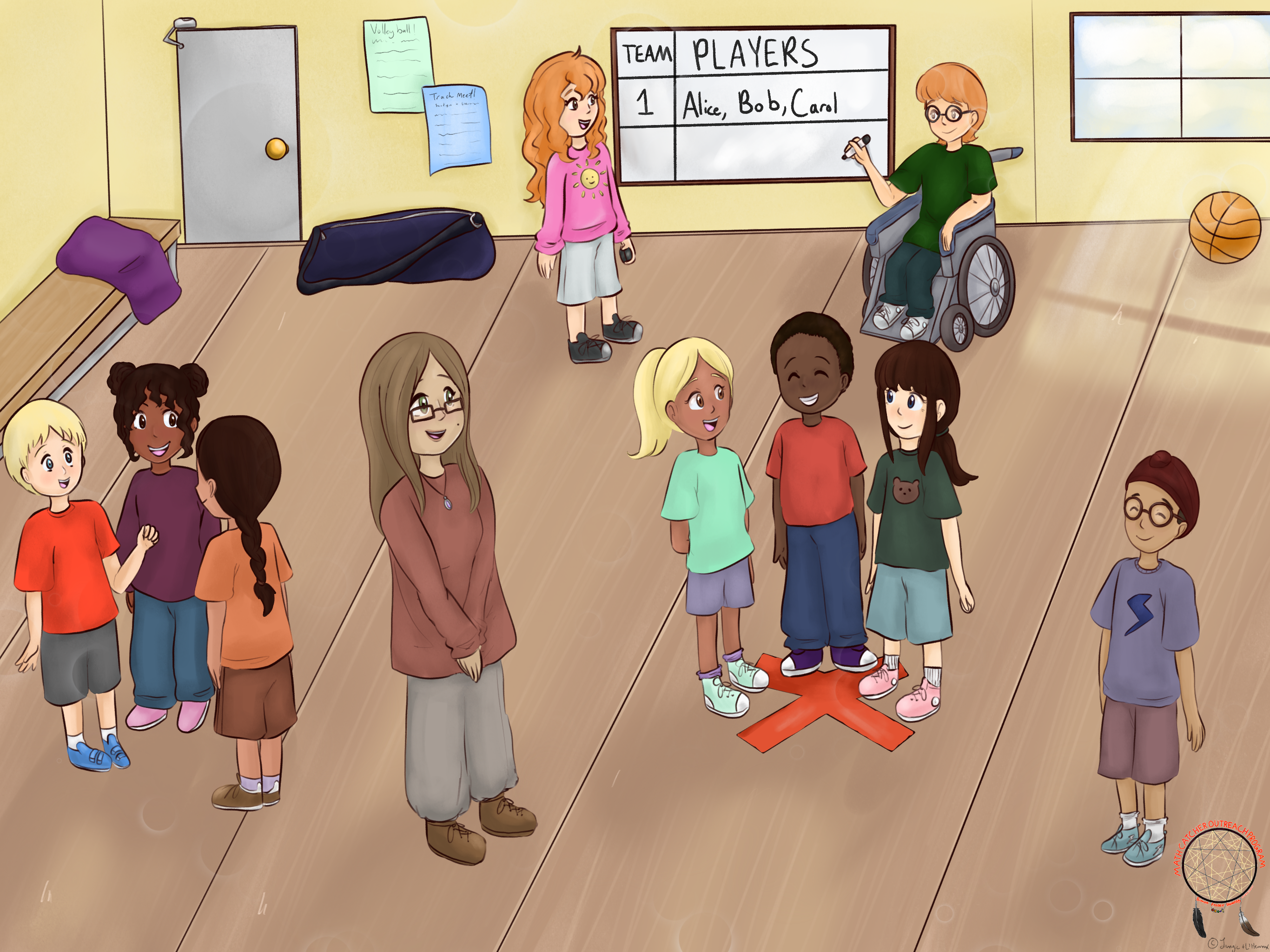To the class: “Thanks! OK, what should we do next?” [The idea is that Dave joins Alice and Bob as a team member and Carol walks away from the marked spot. Maybe a student will suggests this, but maybe the teacher will need to help.]

To Dave and Carol: “Carol, please go back to your place. Dave, please go and join Alice and Bob.” [Recall that Alice and Bob are standing at the marked spot.]

To Erin and Frank: “What should you write on the chart now?”

Frank: “I’ll write the number 2.”

Erin writes:

\begin{equation*} \begin{array}{c|c} \text{Team #} \ \ \ &\ \ \hspace{1cm}\text{Players}\hspace{1cm} \ \ \\ \hline 1&\text{Alice, Bob, Carol} \\ \hline 2&\text{Alice, Bob, Dave} \\ \hline & \\ \hline & \\ \end{array} \end{equation*}

To Erin and Frank: “Excellent job, Erin and Frank!”.

To the class: “What should we do now?” [The class should come to the conclusion that two more teams are possible: Alice, Carol, Dave and Bob, Carol, Dave.]

“So how many different teams can we make?” [The class agrees: Four.]

\begin{equation*} \begin{array}{c|c} \text{Team #} \ \ \ &\ \ \hspace{1cm}\text{Players}\hspace{1cm} \ \ \\ \hline 1&\text{Alice, Bob, Carol} \\ \hline 2&\text{Alice, Bob, Dave} \\ \hline 3&\text{Alice, Carol, Dave} \\ \hline 4&\text{Bob, Carol, Dave} \\ \end{array} \end{equation*}

Ask for questions. Thank Alice, Bob, Carol, Dave, Erin and Frank.

Ask witnesses to describe what happened during the activity.

To the class: “Can you think of another way to count how many teams there are?” [Maybe, “Each time we chose a team, one person was left over. So, for each of the four people, we have one team. So there are four different teams.”]

• Conclusion.

To the class: “Remember that in both activities we started by asking four people to do something similar: One person stayed aside and the remaining three participated in some way.”

• Sitting on one of the three chairs (permutations): You remember that Erin had to be very careful in which order she entered the names in the chart and that we had many different cases.
• Staying around a marked spot (combinations): In this activity, Erin could write the team members’ names in any order she wished. It didn’t matter for our count.

“Can you think about something where it is important to do things in order?” [Possible answers: “entering a password”, “playing music”, “telling a story”, or maybe, “the order of mathematical operations, like addition and multiplication”.]

“Can you think about something where it is not important to do things in order?” [Possible answers: “putting socks on”, “making a fruit salad”, “getting on the school bus” or maybe, “the order in which we add two numbers”.]

“Later in school you will learn words like permutations and combinations and how to calculate the number of permutations and number of combinations very quickly. You will also learn that the part of mathematics that studies permutations and combinations is called combinatorics.”

For some aspects of the Indigenous tradition of witnessing, see the article Learning Humility Through Being a Witness.
• “Let me show you how you can count the number of teams quickly, without writing anything down.”

“This is why I like mathematics: Once you understand what is going on, you can answer many questions with not too much effort.”

“Think about making a team with three players as a task of filling each of three boxes with a name, selected from the six options.” See Figure 3.1.3Figure 3.1.3. Big Circle, Small Number, and Perfect Number are on the court.

We just need to keep in mind that the order in which we list the players’ names does not matter. So we need to make sure that we count each team only once. For example, see Figure 3.1.4. Now we think in this way:Figure 3.1.4. Big Circle, Small Number, and Perfect Number are on the court in both cases - avoid double counting!Figure 3.1.5. Three boxes in a row with six players altogether.
• Step 1: In how many different ways can we put six names into three boxes so that each box contains exactly one name and that each name is used only once? Think for the moment that the boxes are coloured, $\color{blue}{blue}\text{,}$ $\color{green}{green}\text{,}$ and $\color{orange}{yellow}\text{.}$ See Figure 3.1.5. For the $\color{blue}{first}$ $\color{blue}{(blue)}$ $\color{blue}{box}$ we have six choices, for the $\color{green}{second}$ $\color{green}{(green)}$ $\color{green}{box}$ there are five choices (because one name is in the $\color{blue}{blue}$ $\color{blue}{box}$ already) and for the $\color{orange}{third}$ $\color{orange}{(yellow)}$ $\color{orange}{box}$ we have four choices (because two out of six names are already taken). So, there are

\begin{equation*} 6\times5\times4 = 120 \end{equation*}

different ways to put six names into three coloured boxes!

We know that, in this count, each team appears more than one time. For example, see Figure 3.1.6Figure 3.1.6. The same team is counted twice: Big Circle, Small Number, and Perfect Number.
• Step 2. Now we count how many times each team of three appeared in the number 120 that we obtained in Step 1.

In how many different ways can we put three names into three boxes so that each box contains exactly one name and that each name is used only once? Think for the moment that the boxes are coloured, $\color{blue}{blue}\text{,}$ $\color{green}{green}\text{,}$ and $\color{orange}{yellow}\text{.}$ For the $\color{blue}{first}$ $\color{blue}{(blue)}$ $\color{blue}{box}$ we have three choices, for the $\color{green}{second}$ $\color{green}{(green)}$ $\color{green}{box}$ there are two choices (because one name is in the $\color{blue}{blue}$ $\color{blue}{box}$ already) and for the $\color{orange}{third}$ $\color{orange}{(yellow)}$ $\color{orange}{box}$ we have only one name remaining (because two out of three names are already taken). See Figure 3.1.7.Figure 3.1.7. Three boxes in a row with three players altogether.

So, there are

\begin{equation*} 3\times2\times1 = 6 \end{equation*}

different ways to put three names into three coloured boxes!

• Step 3: From step 1, we know that there are 120 different ways the six players can be organized into three boxes. But, this method counts the same team multiple times (as in Figure 3.1.6). From step 2, we know that each team of three players can appear in 6 different arrangements within the three boxes. Since these arrangements all represent the same team, the number of unique teams can be calculated as:

\begin{equation*} 120\div6=20 \end{equation*}

Therefore, there are 20 different teams!

• Ask students to pay attention to mathematics (words, geometrical shapes, games, patterns, numbers). Ask students to pay attention to cultural, particularly Indigenous, aspects of the story. Ask students to make their own puzzle that includes double counting.

### Subsection3.1.7Challenge

Ask students to research Pascal’s triangle.